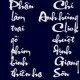Ngô Quốc Anh

December 2, 2008

Differential and Integral Equations through Practical Problems and Exercises (Texts in the Mathematical Sciences)

Filed under: Sách Hay — Ngô Quốc Anh @ 22:27This volume presents a valuable and comprehensive collection of problems and exercises, together with guidelines and worked solutions, of a wide range of the most important commonly occurring differential and integral equations.

The book is divided into two parts. Part I, which comprises eight chapters, contains the problems and exercises, and Part II, having eight corresponding chapters, presents the worked solutions. The various chapters deal, respectively, with: (1) the classical type of differential equations of first and higher order; (2) problems relating to existence and uniqueness theorems; (3) linear differential equations; (4) the method of the Laplace transform; (5) integral and integro-differential equations; (6) numerical and approximation methods; and (7) first-order partial differential equations. The final chapter (8) presents a miscellaneous selection of problems and exercises dealing with the deepest areas of differential, integral and partial differential equations.

Many of the problems discussed are original, and all have been tried and tested in a teaching environment.
This volume provides an invaluable guide to the solving of differential and integral equations and can be recommended to all researchers, students and teachers, whose work involves this fundamental aspect of mathematics.

• Hardcover: 412 pages
• Publisher: Springer; 1 edition (August 31, 1992)
• Language: English
• ISBN-10: 0792318900
• ISBN-13: 978-0792318903
• Product Dimensions: 9.2 x 6.1 x 1 inches

Tôi có bản copy của sách này…

1 Comment »

1.Thay co the gui cho em ban copy do khong ah?
Lien lac qua mail voi em nhe
em cam on ah.

Comment by Quan Bang — September 26, 2009 @ 13:39

This site uses Akismet to reduce spam. Learn how your comment data is processed.